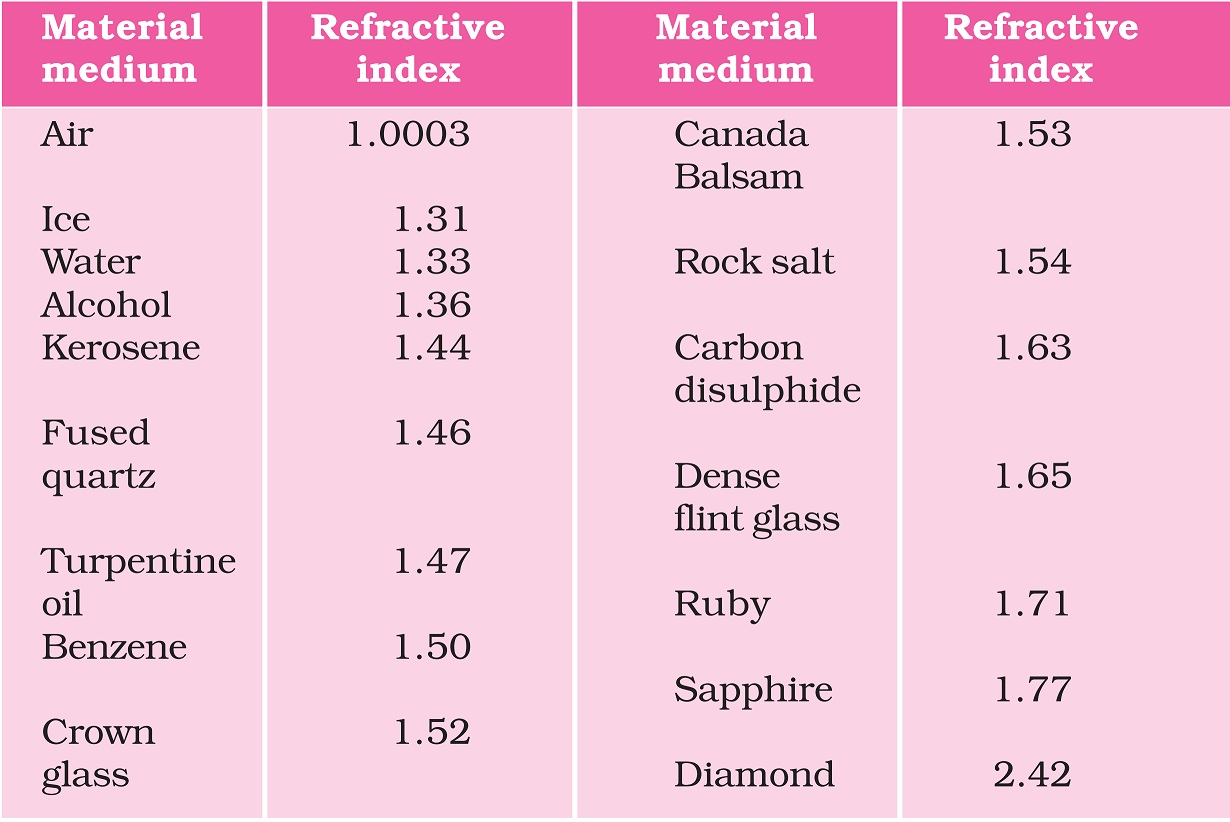Questions from Inside the chapter

Class 10
Chapter 10 Class 10 - Light - Reflection and Refraction (Term 1)

## You are given kerosene, turpentine and water. In which of these does the light travel fastest? Use the information given in Table 10.3

####(adsbygoogle = window.adsbygoogle || []).push({});

We know that,

Refractive index of the medium = Speed of light in air / Speed of light in the medium

Therefore,

Speed of light of the medium = Speed of light in air / Refractive index in the medium

Since Speed of light in air is always constant

Hence,

Speed of light in the medium = Constant/ Refractive index of the medium

Hence, we can say that

Lower refractive index of the medium, the higher is the speed of light.

The refractive index of

• Kerosene is 1.44
• Turpentine is 1.47
• Water is 1.33

Since the refractive index of water is the lowest among the three,

Speed of light in water would be higher than that in kerosene and turpentine.

983 students joined Teachoo Black. What are you waiting for?